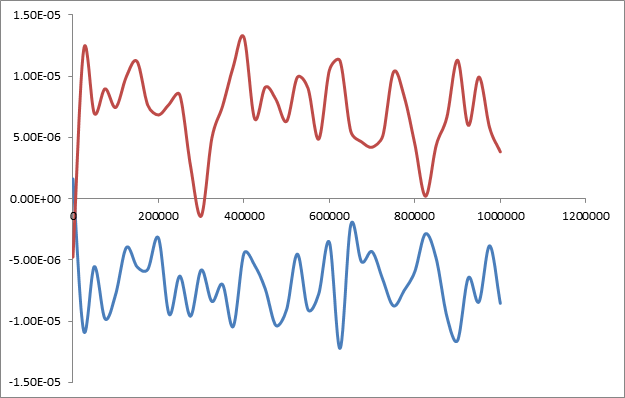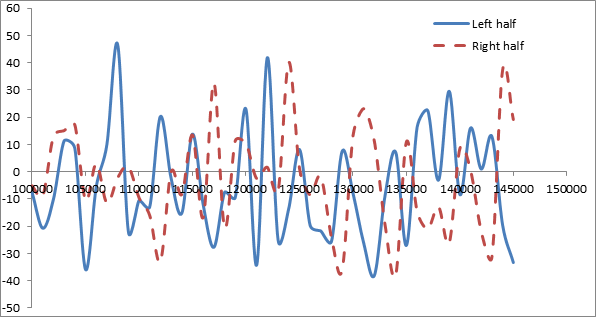# Huge oscillation in the heat flux:heat flux not conserved in NVE

Thanks a lot Dr. Plimpton for your reply. My actual system size is 6720 atoms and 100 Angstroms in the x direction, 2roughly 20 angstroms in other two directions. However following your suggestion I ran a lot of cases with the example code nemd.lmp provided in the lamps website page for calculating thermal conductive using Green Kubo method where the compute heat flux is used.

As I already mentioned I am interested in the heat flux in the system and the thermal conductivity , I changed that script slightly and applied fix heat in the middle and in the two sides. It results in nice steady temperature gradient in the material(Argon I believe). I am supplying energy at the rate 0.02 Kcal/mole/femtosecond (Units real) in the middle and extracting 0.01 Kcal/mole/fs from both ends. NVE is applied for entire box. So the heat flux calculated by compute heat flux in each half of the material should actually correspond the supplied heat flux.

The supplied heat flux (to the left half) in this case:

Energy rate/c-s area

=0.01 Kcal/mole/femtosecond/(21.503 A*21.503 A)

=2.155e-5 Kcal/mole/femtosecond/A^2

Heat flux calculated by compute heat flux (for left half along x direction) in energy*velocity units (averaged over 1 nanosecond runtime)

= -0.06746 Kcal-A/mole/femtosecond

So, dividing by the volume(=21.053 *21.053 *21.053 A^3=9942 A^3)

Heat flux computed in left half

=6.78e-6 Kcal/mole/femtosecond/A^2

Clearly compute heat flux under predicts the flux by an order of magnitude.

The lammps script nemd_test.lmp and resulting thermo logs are attached. I don’t know if this issue relates to system size. My actual system is really big compared to this 21.054 A cube having 256 Ar atoms and I don’t know how well the two values would agree there. The plot of compute heat flux/vol with time is shown below.Thanks and regards,

Souvik